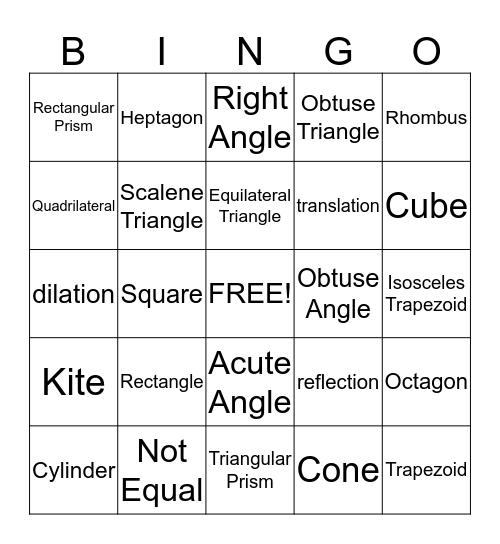# Geometry!This bingo card has a free space and 37 words: Parallelogram, Pentagon, Hexagon, Scalene Triangle, Octagon, Rectangle, Equilateral Triangle, Right Triangle, Rhombus, Isosceles Triangle, Square, Obtuse Triangle, Trapezoid, Isosceles Trapezoid, Quadrilateral, Kite, Circle, Right Angle, Acute Angle, Obtuse Angle, Rectangular Prism, Cube, Cylinder, Triangular Prism, Sphere, Cone, Heptagon, Congruent, Parallel, Equal, Perpendicular, Not Equal, dilation, transformation, reflection, translation and rotation.

## Play Online